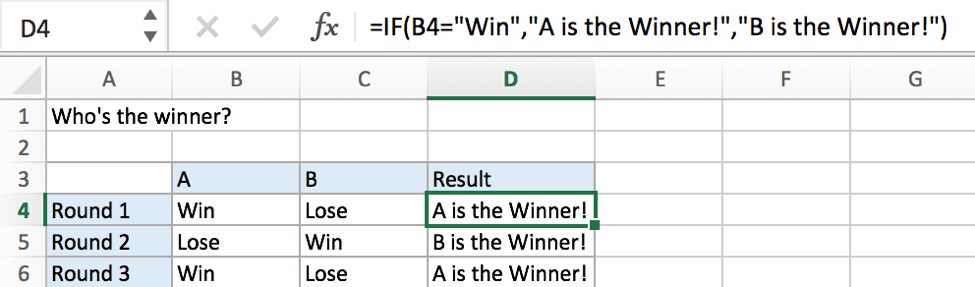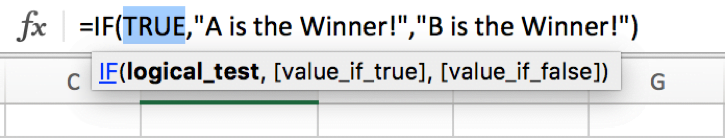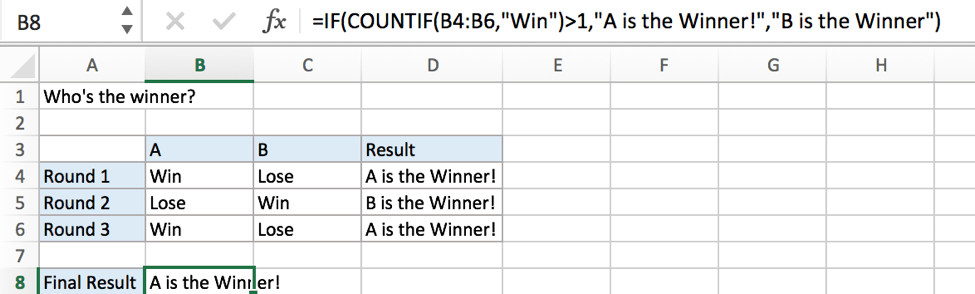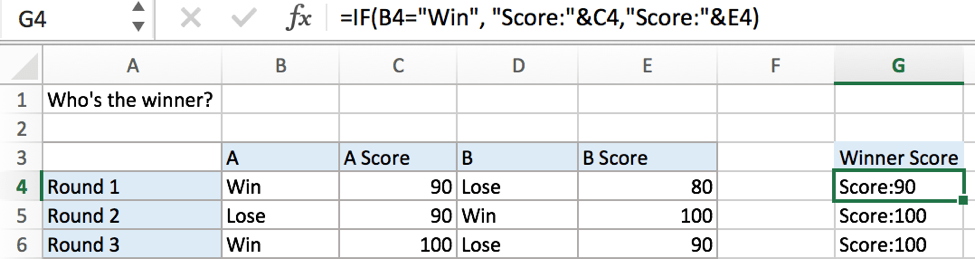# If Cell Equals Certain Text String

IF function is frequently used in Excel worksheet to return you expect “true value” or “false value” based on the result of created logical test. If you want to see if cell equals a certain text string like “Win”, you can build a formula with the help of IF function to return either true result or false result.## FORMULA

To test if cell equals a certain text string, the generic formula is:

=IF(A1=”text”,”true value”,”false value”)

Formula in this example:

=IF(B4=”Win”,”A is the Winner!”,”B is the Winner!”)

## EXPLANATION

In this example, we want to see the results of “A or B who’s the winner” in the three rounds competitions. IF function can handle this case properly.

IF function allows you to create a logical comparison between your value and reference value (for example “A1>0”), and set true value and false value what you expect to return as test results. IF function returns one of the two results based on logical comparison result.

Syntax: IF(logica_test,[value_if_true],[value_if_false])

To test if B4 equals text “Win”, we can directly create a logical comparison B4=“Win”. “Win” should be quoted by double quotes, if missing double quotes, #NAME? error displays instead and it signifies some errors should be corrected in this formula.

In this example, “Win” is detected in B4, logical test result is true.As the result is true, IF function returns true value “A is the Winner!” in C4 properly. On the other side, cell B5 doesn’t equal text “Win”, IF evaluates false value “B is the Winner!” to C5.

In IF, true value and false value can be set as a text, a number or a formula. We set “A is the Winner!” as true result and “B is the Winner” as false result, you can set what you expect to these two values.

More Complicated Example A

To see who is the winner after three rounds of competitions, the winner at least won two among three rounds of competitions, the number of cells “equals “Win”” should be greater than 1. In this case, we can use IF function together with COUNTIF function.More Complicated Example B

You can also change true value or false value to some complicated formula, strings or combinations.### Related Functions

• Excel COUNTIF function
The Excel COUNTIF function will count the number of cells in a range that meet a given criteria. This function can be used to count the different kinds of cells with number, date, text values, blank, non-blanks, or containing specific characters.etc.= COUNTIF (range, criteria)…
• Excel IF function
The Excel IF function perform a logical test to return one value if the condition is TRUE and return another value if the condition is FALSE. The IF function is a build-in function in Microsoft Excel and it is categorized as a Logical Function.The syntax of the IF function is as below:= IF (condition, [true_value], [false_value])….